• 推荐
• 评论
• 收藏

# 步步为营 .NET 设计模式学习笔记 十三、Bridge (桥接模式)

2022-11-22    3063次浏览

<Design Pattern>结构图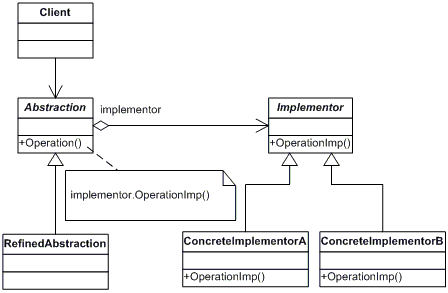• 由抽象化角色和修正抽象化角色组成的抽象化等级结构。
• 由实现化角色和两个具体实现化角色所组成的实现化等级结构。

• 抽象化(Abstraction)角色：抽象化给出的定义，并保存一个对实现化对象的引用。
• 修正抽象化(Refined Abstraction)角色：扩展抽象化角色，改变和修正父类对抽象化的定义。
• 实现化(Implementor)角色：这个角色给出实现化角色的接口，但不给出具体的实现。必须指出的是，这个接口不一定和抽象化角色的接口定义相同，实际上，这两个接口可以非常不一样。实现化角色应当只给出底层操作，而抽象化角色应当只给出基于底层操作的更高一层的操作。
• 具体实现化(Concrete Implementor)角色：这个角色给出实现化角色接口的具体实现。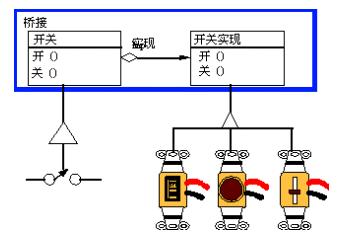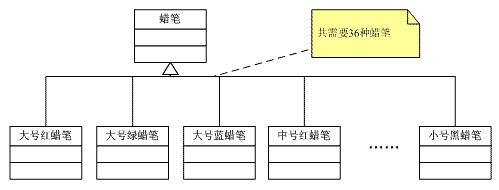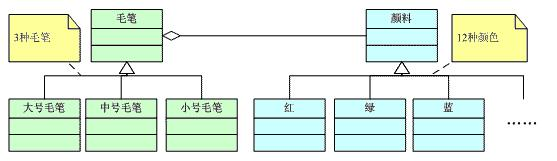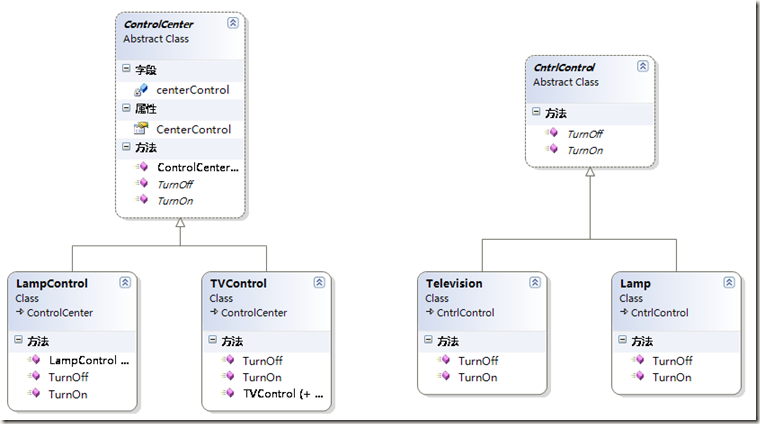`01` `/// `
 `02` `/// control class`
 `03` `/// `
 `04` `public` `abstract` `class` `CntrlControl`
 `05` `{`
 `06` `    ``/// `
 `07` `    ``/// turn on`
 `08` `    ``/// `
 `09` `    ``/// `
 `10` `    ``public` `abstract` `string` `TurnOn();`
 `11`
 `12` `    ``/// `
 `13` `    ``/// turn off`
 `14` `    ``/// `
 `15` `    ``/// `
 `16` `    ``public` `abstract` `string` `TurnOff();`
 `17` `}`

 `01` `public`  `class` `Lamp:CntrlControl`
 `02` `{`
 `03` `    ``public` `override` `string` `TurnOn()`
 `04` `    ``{`
 `05` `        ``return` `"灯亮了"``;`
 `06` `    ``}`
 `07`
 `08` `    ``public` `override` `string` `TurnOff()`
 `09` `    ``{`
 `10` `        ``return` `"灯关了"``;`
 `11` `    ``}`
 `12` `}`

 `01` `public`  `class` `Television:CntrlControl`
 `02` ` ``{`
 `03` `     ``public` `override` `string` `TurnOn()`
 `04` `     ``{`
 `05` `         ``return` `"电视开了"``;`
 `06` `     ``}`
 `07`
 `08` `     ``public` `override` `string` `TurnOff()`
 `09` `     ``{`
 `10` `         ``return` `"电视关了"``;`
 `11` `     ``}`
 `12` ` ``}`

 `01` `/// `
 `02` `/// control center`
 `03` `/// `
 `04` `public` `abstract` `class` `ControlCenter`
 `05` `{`
 `06` `    ``private` `CntrlControl centerControl;`
 `07`
 `08` `    ``public` `CntrlControl CenterControl`
 `09` `    ``{`
 `10` `        ``get`
 `11` `        ``{`
 `12` `            ``return` `centerControl;`
 `13` `        ``}`
 `14` `        ``set`
 `15` `        ``{`
 `16` `            ``centerControl = value;`
 `17` `        ``}`
 `18` `    ``}`
 `19`
 `20` `    ``public` `ControlCenter()`
 `21` `    ``{ }`
 `22`
 `23` `    ``public` `ControlCenter(CntrlControl cntrlControl)`
 `24` `    ``{`
 `25` `        ``this``.centerControl = cntrlControl;`
 `26` `    ``}`
 `27`
 `28` `    ``public` `abstract` `string` `TurnOn();`
 `29`
 `30` `    ``public` `abstract` `string` `TurnOff();`
 `31` `}`

 `01` `public` `class` `LampControl:ControlCenter`
 `02` `{`
 `03` `    ``public` `override` `string` `TurnOn()`
 `04` `    ``{`
 `05` `        ``return` `"我房间的灯控制"``+ CenterControl.TurnOn();`
 `06` `    ``}`
 `07`
 `08` `    ``public` `override` `string` `TurnOff()`
 `09` `    ``{`
 `10` `        ``return` `"我房间的灯控制"` `+ CenterControl.TurnOff();`
 `11` `    ``}`
 `12`
 `13` `    ``public` `LampControl()`
 `14` `    ``{ }`
 `15`
 `16` `    ``public` `LampControl(CntrlControl cntrlControl)`
 `17` `        ``: ``base``(cntrlControl)`
 `18` `    ``{ }`
 `19` `}`

 `01` `public` `class` `TVControl:ControlCenter`
 `02` `{`
 `03` `    ``public` `override` `string` `TurnOn()`
 `04` `    ``{`
 `05` `        ``return` `"客厅的电视控制"``+ CenterControl.TurnOn();`
 `06` `    ``}`
 `07`
 `08` `    ``public` `override` `string` `TurnOff()`
 `09` `    ``{`
 `10` `        ``return` `"客厅的电视控制"` `+ CenterControl.TurnOff();`
 `11` `    ``}`
 `12`
 `13` `    ``public` `TVControl()`
 `14` `    ``{ }`
 `15`
 `16` `    ``public` `TVControl(CntrlControl cntrlControl)`
 `17` `        ``: ``base``(cntrlControl)`
 `18` `    ``{ }`
 `19` `}`

 `01` `public` `partial` `class` `Run : Form`
 `02` `{`
 `03` `    ``public` `Run()`
 `04` `    ``{`
 `05` `        ``InitializeComponent();`
 `06` `    ``}`
 `07`
 `08` `    ``private` `void` `btnRun_Click(``object` `sender, EventArgs e)`
 `09` `    ``{`
 `10`
 `11` `        ``//-------------------------------------`
 `12`
 `13` `        ``ControlCenter lampControl = ``new` `LampControl(``new` `Lamp());`
 `14` `        ``rtbResult.AppendText(``"节能灯控制开始.\n"``);`
 `15` `        ``rtbResult.AppendText(lampControl.TurnOn() + ``"\n"``);`
 `16` `        ``rtbResult.AppendText(lampControl.TurnOff() + ``"\n"``);`
 `17` `        ``rtbResult.AppendText(``"节能灯控制结束.\n\n\n"``);`
 `18` `        ``ControlCenter tvControl = ``new` `TVControl(``new` `Television());`
 `19` `        ``rtbResult.AppendText(``"电视控制开始.\n"``);`
 `20` `        ``rtbResult.AppendText(tvControl.TurnOn() + ``"\n"``);`
 `21` `        ``rtbResult.AppendText(tvControl.TurnOff() + ``"\n"``);`
 `22` `        ``rtbResult.AppendText(``"电视控制结束.\n"``);`
 `23`
 `24` `        ``//-------------------------------------`
 `25` `    ``}`
 `26` `}`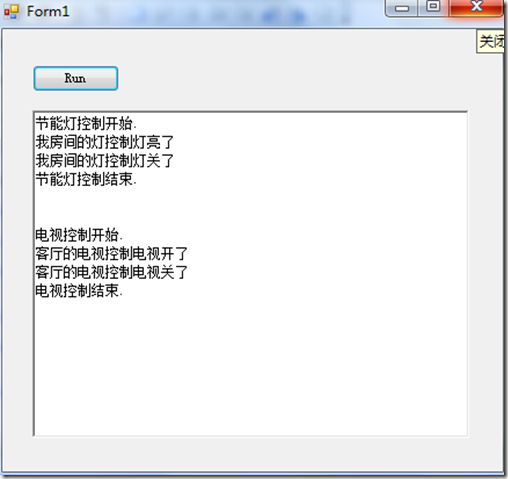1．Bridge模式使用“对象间的组合关系”解耦了抽象和实现之间固有的绑定关系，使得抽象和实现可以沿着各自的维度来变化。

2．所谓抽象和实现沿着各自维度的变化，即“子类化”它们，得到各个子类之后，便可以任意它们，从而获得不同平台上的不同型号。

3．Bridge模式有时候类似于多继承方案，但是多继承方案往往违背了类的单一职责原则（即一个类只有一个变化的原因），复用性比较差。Bridge模式是比多继承方案更好的解决方法。

4．Bridge模式的应用一般在“两个非常强的变化维度”，有时候即使有两个变化的维度，但是某个方向的变化维度并不剧烈——换言之两个变化不会导致纵横交错的结果，并不一定要使用Bridge模式。

5．选择合适的类型作为抽象化角色（第一维度）。

6．抽象化角色和实现化角色通过组合进行关联。

7．抽象和实现不绑定，允许客户端作切换。

1．如果一个系统需要在构件的抽象化角色和具体化角色之间增加更多的灵活性，避免在两个层次之间建立静态的联系。

2．设计要求实现化角色的任何改变不应当影响客户端，或者说实现化角色的改变对客户端是完全透明的。

3．一个构件有多于一个的抽象化角色和实现化角色，系统需要它们之间进行动态耦合。

4．虽然在系统中使用继承是没有问题的，但是由于抽象化角色和具体化角色需要独立变化，设计要求需要独立管理这两者。

5．从代码角度来说，如果类型的继承是处于2个目的（违背单一职责原则）的话可以使用Bridge模式避免过多的子类。

6．从应用角度来说， 如果应用会在多个维度上进行变化，客户端希望两个维度（场景、游戏模式）的对象相对独立，动态耦合（客户端决定哪个场景和哪个游戏模式耦合）的时候可以考虑Bridge模式。

Bridge模式是一个非常有用的模式，也非常复杂，它很好的符合了开放-封闭原则和优先使用对象，而不是继承这两个面向对象原则。

相关知识